Courses

# Thermodynamics & Power Plant - 2

## 20 Questions MCQ Test GATE Mechanical (ME) 2022 Mock Test Series | Thermodynamics & Power Plant - 2

Description
This mock test of Thermodynamics & Power Plant - 2 for GATE helps you for every GATE entrance exam. This contains 20 Multiple Choice Questions for GATE Thermodynamics & Power Plant - 2 (mcq) to study with solutions a complete question bank. The solved questions answers in this Thermodynamics & Power Plant - 2 quiz give you a good mix of easy questions and tough questions. GATE students definitely take this Thermodynamics & Power Plant - 2 exercise for a better result in the exam. You can find other Thermodynamics & Power Plant - 2 extra questions, long questions & short questions for GATE on EduRev as well by searching above.
QUESTION: 1

### The e.m.f. in a thermocouple with the test junction at t°C on gas thermometer scale and reference junction at ice point is given by ε = 0.4 t2 − 15 × 10−4 t3 mv. The millivoltmeter is calibrated at ice and stream points. What will this thermometer read in a place where the thermometer reads 60°C

Solution:

At ice point, when t 0°C, ξ = 0 mV at steam point, when t = 100°C

ξ = 0.4 (100)2 -15 X 10-4 (100)3 = 2500 mV

at         t = 60°C

ξ = 0.4 (60)2- 15 x 10-4 (60)3

= 1116 mV

When gas thermometer reads 60°C, the termocouple will read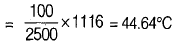QUESTION: 2

### Pv diagram for n moles of an ideal gas is shown in figure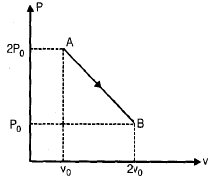Q. The temperature volume graph will be

Solution: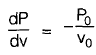∴At A      Pv = 2Povo

At B      Pv = 2Povo

∴  Pv = mRT

∴  TA = TB

which is only in graph (d).

QUESTION: 3

### Find the maximum temperature between A and B

Solution:

From the graph the P-V equation can be written as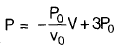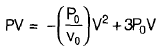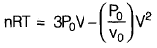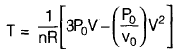for Tmax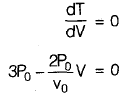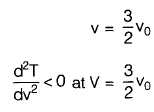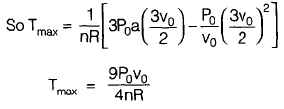QUESTION: 4

An imaginary engine receives heat and does work on a slowly moving piston at such rates that the cycle of operation of 1 kg of working fluid can be represented as a circle 10 cm in diameter on a P-v diagram an which 1 cm = 300 kPa and 1 cm = 0.1 m3/kg. If the heat rejected by engine in a cycle is 1000 kJ/kg of working fluid, what will be its thermal efficiency?

Solution:

As we know that area of cycle on Pv

diagram gives work.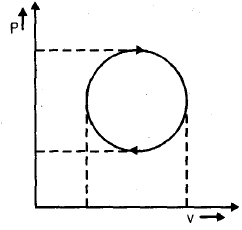So     W = πr2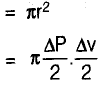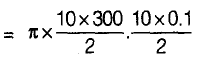= 2355 kJ/kg

∑W = ∑Q

Qreceived  ~  Qrejected = 2355.

Qreceived = 2355 + 1000 3355 kJ/kg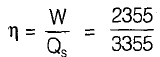= 50.701

QUESTION: 5

Air at 101.325 kPa, 20° C is taken in to a gas turbine power plant at a velocity of 140 m/s through an opening of 0.15 m2 cross-section area. The air is compressed, heated, expanded through a turbine and at 0.18 MPa 150° C through an opening of 0.10 m2 cross-sectional area. The power output is 375 kw. The net amount of heat added to the air in kJ/kg is

Solution: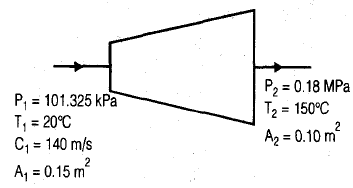specific volume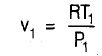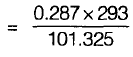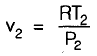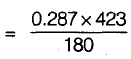v2 = 0.674 m3/kg from mass conservation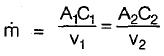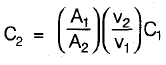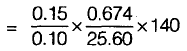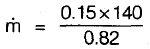= - 25.60 kg/s

Now from SFEE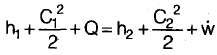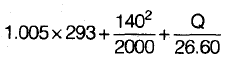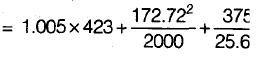Q = 150.41 kJ/kg

QUESTION: 6

A reversible heat engine operates between 600° C and 40° C. This engine drives a reversible refrigerator operating between 40° C and –18° C. Still there is a net work output of 3270 kJ. While the heat received by the engine by 2100 kJ. The cooling effect of refrigerator is

Solution: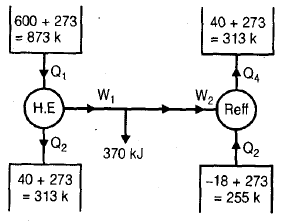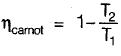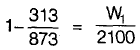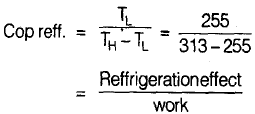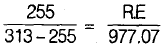R.E = 4295.73 - 4296 kJ

QUESTION: 7

When the outside temperature is –10°C, a residential heat pump must provide 3.5 × 106 kJ per day to dwelling to maintain its temperature at 20°C. If the electricity costs Rs. 2.10 kWh, find the minimum operating cost for each day of operation.

Solution: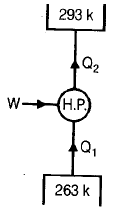Q2 = 3.5 x 106 kJ per day

COP =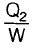for minimum cost

COP =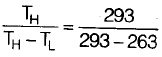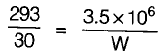W = 0.358x 106 kJ

1 kwh = 3600 kJ

W = 99.44 kwh

cost = 99.44 x 2.10 As

= 208.83 Rs

QUESTION: 8

In a Carnot cycle, heat is supplied at 350° C and rejected at 27° C. The working fluid is water which, while receiving heat, evaporates from liquid at 350° C to steam at 350° C. The associate entropy change is 1.44 kJ/kg K.
Q. If cycle operates on stationary mass of 1 kg of water, work done per cycle and heat supplied will be respectively

Solution: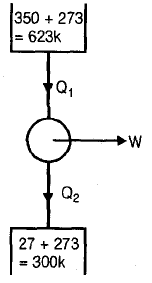T1 = 273 + 350 = 623 k

T2 = 27 + 273 = 300 k

Entropy change = 1.44 kJ/kgk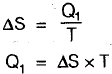= 1.44 x 623 = 897.12 kJ/kg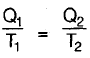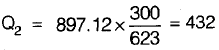W = Q1 Q2

= 897.12-432 = 465.12 kJ/kg

QUESTION: 9

In a Carnot cycle, heat is supplied at 350° C and rejected at 27° C. The working fluid is water which, while receiving heat, evaporates from liquid at 350° C to steam at 350° C. The associate entropy change is 1.44 kJ/kg K.
Q.If the cycle operates in steady flow with a power output of 20 kW, then steam flow rate will be

Solution:

W = 20 kW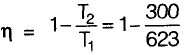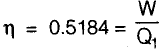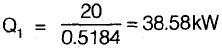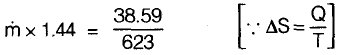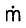= 0.043 kg/s

QUESTION: 10

A gas is compressed reversibly and adiabatically from state ‘a’ to state ‘b’. It is then heated at constant volume to state ‘c’. It is then taken through an isentropic process such that Tc = Td . It is then heated at constant pressure to state ‘e’ such that Tc = Te . Heat is then rejected reversibly from the gas at constant volume till it returns to state ‘a’. Take γ = 1.4.

Q. Ta in terms of Tb and Tc will be

Solution: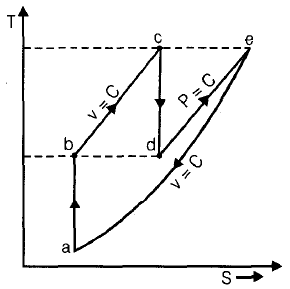Tb = Td

Tc = Tc

For process a to b

Ta Va r-1 = Ta Vb r-1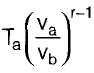For process b to c

Vb = Vc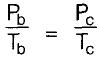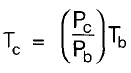QUESTION: 11

A gas is compressed reversibly and adiabatically from state ‘a’ to state ‘b’. It is then heated at constant volume to state ‘c’. It is then taken through an isentropic process such that Tc = Td . It is then heated at constant pressure to state ‘e’ such that Tc = Te . Heat is then rejected reversibly from the gas at constant volume till it returns to state ‘a’. Take γ = 1.4.

Q. If Tb = 555 k and Tc = 8735 k then Ta would be

Solution:
QUESTION: 12

A steam boiler initial contains 5 m3 of steam and 5 m3 of water at 1 MPa. Steam is taken out at constant pressure unit 4m3 of water is left.

Given : at 1 MPa Vf = 0.001127 m3/kg Vg = 0.1944 m3/kg

Q. Mass of steam taken out of the boiler is

Solution:

Initial mass of saturated water and steam in the boiler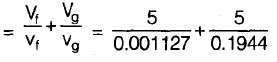= (4.45 x 103 + + 25.70) kg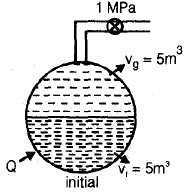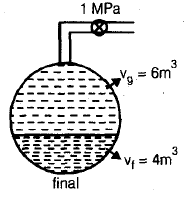Final mass of saturated water and steam =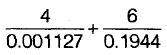= (3.55 x 103 + 30.80) kg Mass of steam taken out to the boiler = (4.45 x 103 + 25.70)-(3.55x 103 + 30.80) (ms = 894.9 kg)

From energy balance :

Initial energy stored in saturated water and steam + heat transferred from external source = final energy storted in saturated water and steam + energy leaving with steam

(4.45 x 103 + 761.68) + (25.70 x 2583.6)+Q = (3.55x 103 + 761.68) + (30.8 x 2583.6) + 894.9x2778.1

Q = 1752.676 MJ

QUESTION: 13

A steam boiler initial contains 5 m3 of steam and 5 m3 of water at 1 MPa. Steam is taken out at constant pressure unit 4m3 of water is left.

Given : at 1 MPa Vf = 0.001127 m3/kg Vg = 0.1944 m3/kg

Q. Heat transfer during the process will be

Solution:

Initial mass of saturated water and steam in the boiler= (4.45 x 103 + + 25.70) kgFinal mass of saturated water and steam == (3.55 x 103 + 30.80) kg Mass of steam taken out to the boiler = (4.45 x 103 + 25.70)-(3.55x 103 + 30.80) (ms = 894.9 kg)

From energy balance :

Initial energy stored in saturated water and steam + heat transferred from external source = final energy storted in saturated water and steam + energy leaving with steam

(4.45 x 103 + 761.68) + (25.70 x 2583.6)+Q = (3.55x 103 + 761.68) + (30.8 x 2583.6) + 894.9x2778.1

Q = 1752.676 MJ

QUESTION: 14

A platinum wire is used as a resistance thermometer the wire resistance was found to be 20 ohm and 36 ohm at ice point and steam point respectively and 40 ohm at melting point of antimony 630.50°C.
Find the resistance of wire of 70°C. If the resistance varies with temperature by the relation R = R0 (1 + at + b2)

Solution:

R = Ro (1 + at + bt2)

at ice point, t = 0

20 = R0 (1 + a x 0 + b x 0) Ro = 20

at steam point t = 1000C

36 = 20 [1 + 100 a + 104 b]

1 + 100a + 104 b = 1.8

100a + 10000 b = 0.8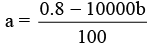at t = 630.50 C

40 = 20 [1 + 630.5a + (630.5)2b]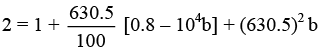1 = 5.04 - 63050 b + 397530.25 b

b = - 1.2 x 10-5 a = 9.2 x 10-3 so at 700° C

R = 20 [1 + 9.2 x 10-3 x 700 - 1.2 x 10-5 (700)2]

= 31.2 ohm

*Answer can only contain numeric values
QUESTION: 15

A water chilling plant remove heat from the water at a rate of 42 MJ/h. Heat leaks in to the water from surrounding at an average rate of 4.2 MJ/h. The time required for cooling a batch for 1500 kg of water from 40° C to 10° C, is _________ hours. (C p = water 4.2 kJ/kgk)

Solution:

Total heat removed from water = (42 – 4.2) MJ/h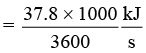= 10.5 kJ / s

This heat removed will decrease the temperature of water

10.5 × t = mCP ∆ T

= 1500 × 4.2 × 30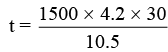= 18000 seconds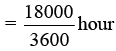= 5 hours

QUESTION: 16

In a vessel, 10 kg of O2 is heated in a reversible now flow, constant volume process so that the pressure of O2 is increased two times of initial value. If the initial temperature is 20°C what will be the internal energy change (Cv = 0.65 kJ/kg k).

Solution:

m = 10 kg, P1 = P, P2 = 2p
T1 = 200 C
v = constant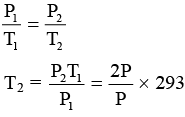T2 = 586 k

∆u = mc ∆T = 10 × 0.65 (586 − 293)
= 1904.5 kJ

QUESTION: 17

Suppose 1g of water vaporizes isobarically at atmospheric pressure (1.01 × 105 Pa). Its volume in liquid state is 1 cm3 and in vapour state is 1671 cm3. Find the change in internal energy of system. (Latent heat of vaporization = 2.96 × 196 J/kg).

Solution:

w = P 0 (vf – vi )
= 1.01 × 105 [1671 × 10–6 – 1 × 10–6]
= 169 J
Q = mL = 1 × 10–3 [2.26 × 106)
= 2260 J
∆U = Q – w = 2260 – 169
= 2091 Joule

*Answer can only contain numeric values
QUESTION: 18

Air at a flow rate of 1 kg/s enters the nozzles of a jet engine at a temperature of 200 kPa and at a velocity of 50 m/s. It leaves nozzle at a temperature of 650 k and pressure of 100 kPa. Heat loss at a constant rate of 25 kw takes place from the nozzle to the surroundings. Assume air to behave as ideal gas with the constant pressure specific heat values of 1.005 kJ/kg and neglect potential energy change, the exit velocity of air is _________ m/s.

Solution: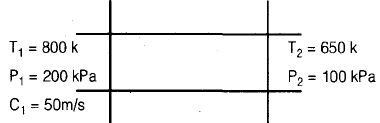m = 1 kg/s
Q = –25 Kw
Cp = 1.005 kJ/kgk
from SFEE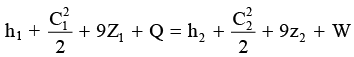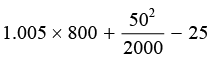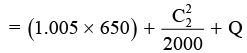C2 = 503.98 m/s
= 504 m/s

QUESTION: 19

A heat pump working on the Carnot cycle takes in heat from a reservoir at 5°C and delivers heat to a reservoir at 60°C. The heat pump is driven by a reversible heat engine which takes heat to a reservoir at 60° C. The reversible heat engine also drives a machine that absorbs 30 kW. If the heat pump extracts 17 kJ/s from the 5°C reservoir, then

Q. Determine the rate of heat supply from 8400° C source

Solution: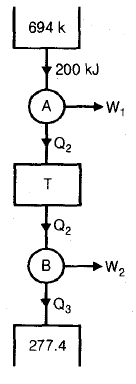QUESTION: 20

A heat pump working on the Carnot cycle takes in heat from a reservoir at 5°C and delivers heat to a reservoir at 60°C. The heat pump is driven by a reversible heat engine which takes heat to a reservoir at 60° C. The reversible heat engine also drives a machine that absorbs 30 kW. If the heat pump extracts 17 kJ/s from the 5°C reservoir, then

Q. Determine the rate of heat rejection to 60° C sink

Solution: